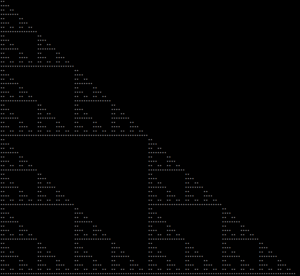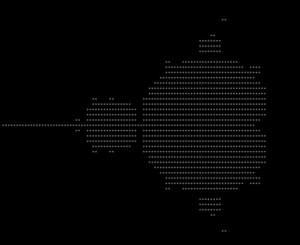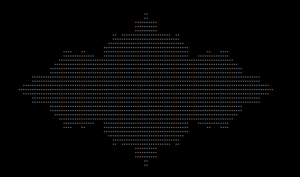# Generating Fractals with Postgres: Escape-Time Fractals

This post is based on half of a talk I gave at !!con. If you want to watch the talk, you can find it here.

One not too well known aspect of SQL is that it’s Turing complete. This means any program you can write in a general purpose programming language, you can also write in SQL. In this post, I’ll show you how you can use some of the dark corners of SQL in order to generate fractals. We’ll ultimately wind up with a set of queries which will allow us to generate an entire class of fractals known as the “escape-time fractals”.

## Common Table Expressions

Before we begin, I’m going to go over one very important SQL feature that I’m going to use a ton throughout this post. That feature is known as CTEs (common table expressions). CTEs effectively give you a way to define what are effectively variables in SQL. They are really nice for large SQL queries because they let you break down the query into smaller pieces.

To define a CTE, you use the WITH clause. Here’s a simple example of a CTE that counts how many numbers there are from 5 to 10

To execute this query Postgres first runs the query

After running that query Postgres aliases the results of that query as numbers. That means from then on, you use use numbers as if it were a table. The numbers “table” has in it the results of the previous query. This is just like we are saving the results of the generate_series query into the variable numbers. As I said before, this is really nice because it gives you a way to pull out portions of a large query into separate components.

Now that we’ve gone over CTEs, we can get to the meat of the post, generating fractals.

## The Sierpinski Triangle

There are many different ways to generate the Sierpinski triangle. One that’s especially convenient is based on bitwise arithmetic. If you take a grid and fill in all the cells where the bitwise AND of the row number and column number is zero, you get the Sierpinski triangle. It’s not intuitive, but here’s a few examples demonstrating that this approach works:

We can see that as we increase the size of the grid, we get a better resolution version of the Sierpinski triangle.

To execute this algorithm in SQL, we can write a three part SQL query:

• First, we use the generate_series function and CROSS JOIN to produce one row per cell in the grid.
• Next, we use CASE WHEN (analogous to if-else in any other programming language) to assign a string to each row based on the bitwise AND of the row number and column number.
• Finally, we concatenate all the cells together using the string_agg function.

The first part is relatively straightforward. We use two calls to generate_series, one to generate the row numbers and a second to generate the column numbers. We can then use a CROSS JOIN to generate every pair of row number and column number. This gives us our grid:

The next part is also straightforward. For each cell we assign a string based on the bitwise AND of the row number and column number. Let’s assign ‘**’ to cells that are part of the triangle and ‘  ‘ to cells that are not. In SQL this looks something like this:

Now that we’ve got our marked points, the only thing left to do is to combine all the strings together. We can do this with two calls to the string_agg function. string_agg is an aggregation function that concatenates all the given strings together. We first use it on the values from each row to generate one string for each row. We then use it a second time to concatenate all the rows together. In the end this looks like this:## The Mandelbrot Set

Now that we’ve got the Sierpinski triangle, the next question is whether or not we can generalize this code. If you look closely you will notice there’s one nice place where we can generalize the code. In the current version, we use bitwise AND to determine whether or not the cell should be part of the fractal:

We can actually replace that test with any test we want. This means if, for a given fractal, we can come up with an easy test to determine whether or not a cell should be part of the fractal, we can produce a SQL query that generates it!

One well known fractal that has this property is the Mandelbrot set. You can test whether or not a point is in the Mandelbrot set by repeatedly running the following formula over each point (i in the formula is the imaginary number):

If z[n] does not diverge to infinity as n increases, the point is part of the Mandelbrot set. This is usually implemented by running the formula for a fixed number of iterations and checking whether z[n] has crossed some threshold.

To implement this test in SQL, we will need a way to express iteration. One way of doing iteration in SQL is what’s known as a “Recursive CTE“. A recursive CTE has similar syntax to a regular CTE, but is actually completely different. The rough idea with a recursive CTE is that you provide an “initial query” and a “recursive query”. It ultimately looks something like this:

Postgres executes a recursive CTE by first executing the initial query. Postgres takes the results of the initial query and feeds those into the recursive query. Postgres then takes the results of that and feeds them back into the recursive query. Postgres keeps doing this until one of the queries returns no results. At that point the result of the entire recursive CTE is the concatenation of the results of every query that ran as part of executing the CTE. As a specific example, here’s a query that generates the numbers one through five:

To execute the above query, Postgres first executes the initial query SELECT 1. This simply returns the value 1. Postgres then feeds that into the recursive query which selects i+1 as long as i is less than five. This first returns 2, then 3, then 4, and finally 5, at which point the recursive query returns no results. At this point the result of the recursive CTE is the values 1 through 5.

With our new knowledge of recursive CTEs, we can now write a query that can test whether or not a given point is part of the Mandelbrot set. First we use a recursive CTE to evaluate the formula for every point one thousand times. That looks something like the following query that makes use of complex arithmetic:

We can then test whether a point is part of the Mandelbrot set with the following predicate:

If you take the query for producing the Sierpinski triangle and replace the part for testing the bitwise AND with the test for the Mandelbrot set, you get the following query:## Escape-Time Fractals

As it turns out there is a class of fractals based on this idea of repeatedly evaluating a function at each point in a space. This class of fractals is known as the “escape-time fractals”. We only need to slightly modify our query to generate the Mandelbrot set and we can easily produce tons of different escape-time fractals. There’s an interesting set of these fractals known as the Julia Sets. These are based on running the following formula for a given function f(z):

One particularly interesting example is based on the following formula (where φ is the golden ratio):

If we modify our query for generating the Mandelbrot Set to run this formula instead:

We get the following fractal:So based on the query we wrote, we can generate tons of different fractals by just changing a few different values in our query!